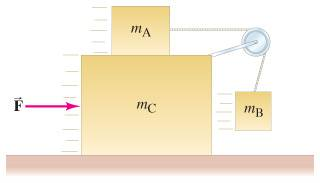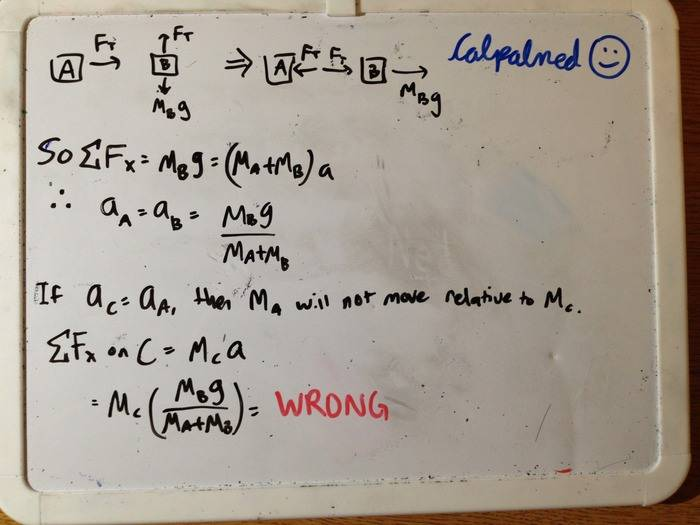# Find force so that the mass does not move

## Homework Statement[/B]
Determine a formula for the magnitude of the force F exerted on the large block (mC) in the figure so that the mass mA does not move relative to mC. Ignore all friction. Assume mB does not make contact with mC.

F = ma

## The Attempt at a SolutionThank you all so much.

NascentOxygen
Staff Emeritus
The blocks stay fixed in their relative positions. So to accelerate the whole assemblage: F = (mA + mB + mC).a

Does the textbook provide the correct answer? I'm not sure whether it's accurate enough to say the tension in the rope simply overcomes B's weight; you may need to acknowledge that the tension also provides B's horizontal acceleration. (Hence the rope's deviation from the vertical.)

Last edited:
Chestermiller
Mentor

## Homework Statement[/B]
Determine a formula for the magnitude of the force F exerted on the large block (mC) in the figure so that the mass mA does not move relative to mC. Ignore all friction. Assume mB does not make contact with mC.

F = ma

## The Attempt at a SolutionThank you all so much.
You almost had it right, but not quite. The key to doing this problem correctly is to get the force balance on mass B correct.

Let T be the tension in the string and let θ represent the angle that the string attached at mass B makes with the vertical (i.e., the string is not vertical). In terms of T and θ, what is the horizontal component of the string tension on mass B? In terms of T and θ, what is the vertical component of the string tension on mass B? How does the horizontal component of acceleration of mass B compare with the acceleration a of mass A? What is the vertical component of the acceleration of mass B? In terms of T, θ, mB, and a, what is the horizontal force balance on mass B? In terms of T, θ, mB, what is the vertical force balance on mass B. In terms of T, mA, and a, what is the horizontal force balance on mass A?

If you did all this correctly, you will now have three equations in three unknowns: T, θ, and a. Solve these equations for the acceleration a.

By the way, when you finish with all this, you will find that you get the same answer that NascentOxygen gave.

Chet

Last edited: